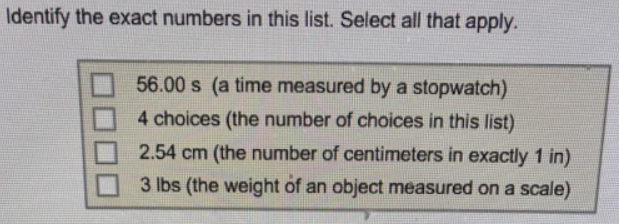# Problem: Identify the exact numbers in this list. Select all that apply. (a) 56.00 s (a time measured by a stopwatch) (b) 4 choices (the number of choices in this list) (c) 2.54 cm (the number of centimeters in exactly 1 in) (d) 3 lbs (the weight of an object measured on a scale)

###### FREE Expert Solution

Exact numbers are defined to be definite and usually expressed in whole numbers.

81% (452 ratings)###### Problem Details

Identify the exact numbers in this list. Select all that apply.

(a) 56.00 s (a time measured by a stopwatch)

(b) 4 choices (the number of choices in this list)

(c) 2.54 cm (the number of centimeters in exactly 1 in)

(d) 3 lbs (the weight of an object measured on a scale)